Displayed Output

The output of PROC CALIS includes the following:

• a list of basic modeling information such as: the data set, the number of records read and used in the raw data set, the number of observations assumed by the statistical analysis, and the model type. When a multiple-group analysis is specified, the groups and their corresponding models are listed. This output assumes at least the PSHORT option.

• a list of all variables in the models. This output is displayed by default or by the PINITIAL option. It will not be displayed when you use the PSHORT or the PSUMMARY option.

Depending on the modeling language, the variable lists vary, as shown in the following:

• FACTOR: a list of the variables and the factors

• LINEQS, PATH, and RAM: a list of the endogenous and exogenous variables specified in the model

• LISMOD: a list of-,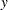-,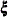-, and
- variables specified in the model

• MSTRUCT: a list of the manifest variables specified in the model

• initial model specification. This output is displayed by default or by the PINITIAL option. It will not be displayed when you use the PSHORT or the PSUMMARY option.

Depending on the modeling language, the sets of output vary, as shown in the following:

• FACTOR: factor loading matrix, factor covariance matrix, intercepts, factor means, and error variances as specified initially in the model. The initial values for free parameters, the fixed values, and the parameter names are also displayed.

• LINEQS: linear equations, variance and covariance parameters, and mean parameters as specified initially in the model. The initial values for free parameters, the fixed values, and the parameter names are also displayed.

• LISMOD: all model matrices as specified initially in the model. The initial values for free parameters, the fixed values, and the parameter names are also displayed.

• MSTRUCT: initial covariance matrix and mean vectors, with parameter names and initial values displayed.

• PATH: the path list, variance and covariance parameters, intercept and mean parameters as specified initially in the model. The initial values for free parameters, the fixed values, and the parameter names are also displayed.

• RAM: a list of parameters, their types, names, and initial values.

• mean and standard deviation of each manifest variable if you specify the SIMPLE option, as well as skewness and kurtosis if the DATA= data set is a raw data set and you specify the KURTOSIS option.

• various coefficients of multivariate kurtosis and the numbers of observations that contribute most to the normalized multivariate kurtosis if the DATA= data set is a raw data set and the KURTOSIS option is used or you specify at least the PRINT option. See the section Measures of Multivariate Kurtosis for more information.

• covariance coverage, variable coverage, average coverage of covariances and means, rank orders of the variable (mean) and covariance coverage, most frequent missing patterns in the input data set, and the means of the missing patterns when there are incomplete observations (with some missing values in the analysis variables) in the input raw data set and when you use METHOD=FIML or METHOD=LSFIML for estimation.

• covariance or correlation matrix to be analyzed and the value of its determinant if you specify the output option PCORR or PALL. A zero determinant indicates a singular data matrix. In this case, the generalized least squares estimates with default weight matrix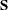and maximum likelihood estimates cannot be computed.

• the weight matrix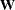or its inverse is displayed if GLS, WLS, or DWLS estimation is used and you specify the PWEIGHT or PALL option.

• initial estimation methods for generating initial estimates. This output is displayed by default. It will not be displayed when you use the PSHORT or the PSUMMARY option.

• vector of parameter names and initial values and gradients. This output is displayed by default, unless you specify the PSUMMARY or NOPRINT option.

• special features of the optimization technique chosen if you specify at least the PSHORT option.

• optimization history if at least the PSHORT option is specified. For more details, see the section Use of Optimization Techniques.

• specific output requested by options in the NLOPTIONS statement; for example, parameter estimates, gradient, constraints, projected gradient, Hessian, projected Hessian, Jacobian of nonlinear constraints, estimated covariance matrix of parameter estimates, and information matrix. Note that the estimated covariance of parameter estimates and the information matrix are not printed for the ULS and DWLS estimation methods.

• fit summary table with various model fit test statistics or fit indices, and some basic modeling information. For the listing of fit indices and their definitions, see the section Overall Model Fit Indices. Note that for ULS and DWLS estimation methods, many of those fit indices that are based on model fitare not displayed. See the section Overall Model Fit Indices for details. This output can be suppressed by the NOPRINT option.

• fit comparison for multiple-group analysis. See the section Individual Fit Indices for Multiple Groups for the fit indices for group comparison. This output can be suppressed by the NOPRINT option.

• the predicted covariance matrix and its determinant and mean vector, if you specify the output option PCORR or PALL.

• residual and normalized residual matrix if you specify the RESIDUAL option or at least the PRINT option. The variance standardized or asymptotically standardized residual matrix can be displayed also. The average residual and the average off-diagonal residual are also displayed. Note that normalized or asymptotically standardized residuals are not applicable for the ULS and DWLS estimation methods.

See the section Residuals for more details.

• rank order of the largest normalized residuals if you specify the RESIDUAL option or at least the PRINT option.

• bar chart of the normalized residuals if you specify the RESIDUAL option or at least the PRINT option.

• plotting of smoothed density functions of residuals if you request ODS Graphics by the PLOTS= option.

• equations of linear dependencies among the parameters used in the model specification if the information matrix is recognized as singular at the final solution.

• the estimation results and the standardized results. Except for ULS or DWLS estimates, the approximate standard errors andvalues are also displayed. This output is displayed by default or if you specify the PESTIM option or at least the PSHORT option.

Depending on the modeling language, the sets of output vary, as shown in the following:

• FACTOR: factor loading matrix, rotation matrix, rotated factor loading matrix (if rotation requested), factor covariance matrix, intercepts, factor means, and error variances in the model. Factor rotation matrix is printed for the unstandardized solution.

• LINEQS: linear equations, variance and covariance parameters, and mean parameters in the model.

• LISMOD: all model matrices in the model.

• MSTRUCT: covariance matrix and mean vectors.

• PATH: the path list, variance and covariance parameters, intercept and mean parameters.

• RAM: a list of parameters, their types, names, and initial values.

• squared multiple correlations table which displays the error variance, total variance, and the squared multiple correlation of each endogenous variable in the model. The total variances are the diagonal elements of the predicted covariance matrix. This output is displayed if you specify the PESTIM option or at least the PSHORT option.

• the total determination of all equations, the total determination of the latent equations, and the total determination of the manifest equations if you specify the PDETERM or the PALL option. See the section Assessment of Fit for more details. If you specify subsets of variables in the DETERM statements, the corresponding determination coefficients will also be shown. If one of the determinants in the formula for computing the determination coefficient is zero, the corresponding coefficient is displayed as the missing value '.'.

• the matrix of estimated covariances among the latent variables in the model if you specify the PLATCOV option or at least the PRINT option.

• the matrix of estimated covariances between latent and manifest variables in the model if you specify the PLATCOV option or at least the PRINT option.

• the vector of estimated means for the latent and manifest variables in the model if you specify the PLATCOV option or at least the PRINT option.

• the matrix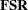of latent variable scores regression coefficients if you specify the PLATCOV option or at least the PRINT option. Thematrix is a generalization of Lawley and Maxwell (1971, p. 109) factor scores regression matrix,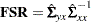where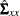is the
predicted covariance matrix among manifest variables and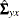is the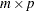matrix of the predicted covariances between latent and manifest variables, withbeing the number of manifest variables, andbeing the number of latent variables. You can multiply the observed values by this matrix to estimate the scores of the latent variables used in your model.

• stability coefficient of reciprocal causation if you request the effect analysis by using the EFFPART or TOTEFF option, and you must not use the NOPRINT option.

• the matrices for the total, direct, and indirect effects if you specify the EFFPART or TOTEFF option or at least the PRINT option, and you must not use the NOPRINT option. Unstandardized and standardized effects are printed in separate tables. Standard errors for the all estimated effects are also included in the output. Additional tables for effects are available if you request specialized effect analysis in the EFFPART statements.

• the matrix of rotated factor loadings and the orthogonal transformation matrix if you specify the ROTATE= and PESTIM options or at least the PSHORT options. This output is available for the FACTOR models.

• factor scores regression matrix, if you specify the PESTIM option or at least the PSHORT option. The determination of manifest variables is displayed only if you specify the PDETERM option.

• univariate Lagrange multiplier indices if you specify the MODIFICATION (or MOD) or the PALL option. The value of a Lagrange multiplier (LM) index indicates the approximate drop inwhen the corresponding fixed parameter in the original model is freely estimated. The corresponding probability (with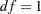) and the estimated change of the parameter value are printed. Ranking of the LM indices is automatically done for prescribed parameter subsets of the original model. The LM indices with greatest improvement ofmodel fit appear in the beginning of the ranking list. Note that LM indices are not applicable to the ULS and the DWLS estimation methods. See the section Modification Indices for more detail.

• matrices of univariate Lagrange multiplier (LM) indices if you specify the MODIFICATION (or MOD) or the PALL option, and the LMMAT option in the LMTESTS statement. These matrices are predefined in PROC CALIS, or you can specify them in the LMTESTS statements. If releasing a fixed parameter in the matrix would result in a singular information matrix, the string 'Singular' is displayed instead of the Lagrange multiplier index. If a fixed entry in the matrix is restricted by the model (for example, fixed ones for coefficients associated with error terms) or being excluded in the specified subsets in the LMTESTS statement, the string 'Excluded' is displayed. Note that matrices for LM indices are not printed for the ULS and the DWLS estimation methods. See the section Modification Indices for more detail.

• univariate Lagrange multiplier test indices for releasing equality constraints if you specify the MODIFICATION (or MOD) or the PALL option. Note that this output is not applicable to the ULS and the DWLS estimation methods. See the section Modification Indices for more detail.

• univariate Lagrange multiplier test indices for releasing active boundary constraints specified by the BOUNDS statement if you specify the MODIFICATION (or MOD) or the PALL option. Note that this output is not applicable to the ULS and the DWLS estimation methods. See the section Modification Indices for more detail.

• the stepwise multivariate Wald test for constraining estimated parameters to zero constants if the MODIFICATION (or MOD) or the PALL option is specified and the univariate probability is greater than the value specified in the PMW= option (default PMW=0.05). Note that this output is not applicable to the ULS and the DWLS estimation methods. See the section Modification Indices for more details.